What's New Table of Contents# What's New in SAS/QC 9.0 and 9.1

## Overview

SAS/QC now includes the ANOM procedure for analysis of means, a graphical and statistical method for comparing a set of means to determine whether any of them are significantly different from the overall mean. There are also a number of enhancements to the existing CAPABILITY, OPTEX, RELIABILITY, and SHEWHART procedures.

The ADX Interface for Design of Experiments is a guided point-and-click solution for engineers, scientists, statisticians, and other researchers who collaboratively design, analyze, and interpret experiments to improve industrial processes and products. The ADX Interface supports a variety of designs, including two-level, mixed level, response surface, mixture, optimal, and split-plot.

The ADX Interface now enables you to:

• create general factorial designs with factors having up to nine levels
• create two-level full factorial and minimum aberration fractional factorial generalized split-plot designs
•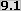delete inactive factors and project a fractional-factorial design to a higher-resolution design
•join the means in a box plot
•show clear and aliased effects in the alias structure
•display confidence intervals in the response calculator and experiment report
•honor block structure in a blocked design during design randomization

The ADX Interface is documented in the book Getting Started with the SAS 9 ADX Interface for Design of Experiments.

## ANOM ProcedureThe ANOM procedure produces analysis of means (ANOM) charts for identifying group means that differ significantly from the overall mean. An ANOM chart is similar to a control chart, with a process variable statistic (such as a mean) plotted versus a classification or group variable. Decision limits on the chart are used to determine which of the group means are significantly different.

ANOM can be used as an alternative to analysis of variance (ANOVA) in the fixed effects situation. ANOM differs from ANOVA in that it identifies the groups that are different; ANOVA only determines whether a significant difference exists. ANOM has the additional advantage of a convenient graphical representation and lends itself to quality improvement applications in which the end user has limited background in statistics.

PROC ANOM can be applied to both variables and attribute data, and to equal and unequal sample sizes.

## CAPABILITY Procedure

You can now juxtapose displays, including box-and-whisker plots, dot plots, and carpet plots, with histograms as aids for visualizing the distribution of process data.

The following options are new in the HISTOGRAM statement:

• The BMCBOXFILL= option specifies the fill color for a box-and-whisker plot in the bottom margin. By default, the box-and-whisker plot is not filled.
• The BMCFRAME= option specifies the color for filling the frame of a bottom margin plot. By default, this area is not filled.
• The BMCOLOR= option specifies the color of the dot plot, carpet
• plot, or the outline of a box-and-whisker plot in the bottom margin.
• The BMMARGIN= option specifies the height of a bottom margin plot.
• The BMPLOT= option produces a box-and-whisker plot, dot plot, or carpet plot along the bottom margin of a histogram. A box-and-whisker plot gives a summary of the data distribution that a histogram alone does not provide. A dot plot or carpet plot shows the distribution of individual observations.

The following options are new in the COMPHIST and HISTOGRAM statements:

• The FRONTREF option causes reference lines to be drawn in front of histogram bars.
• The LOWER= suboption in the KERNEL option specifies lower bounds for fitted kernel density estimates.
• The UPPER= suboption in the KERNEL option specifies upper bounds for fitted kernel density estimates.CLASS= variables specified in the COMPHIST statement can now have values longer than 16 characters.

## OPTEX Procedure

The OPTEX procedure now enables you to control how CLASS variables are modeled, by specifying options for the ordering of levels and the parameterization of design matrix columns associated with the levels. In addition, the default parameterization has been changed to be orthogonal, providing efficiency values and parameter variances that are easier to interpret and to compare.

The following options are new in the CLASS statement:

• The DESCENDING option reverses the sorting order of the classification levels.
• The ORDER= option specifies how the classification levels are sorted.
• The PARAM= option specifies the parameterization method for the design matrix columns associated with classification levels.
• The REF= option specifies the reference level for effect or reference parameterization.

## RELIABILITY Procedure

• The MCFPLOT statement has been enhanced to allow recurrence and censoring ages to be grouped into intervals.
• The INTERPOLATE= option, in the MCFPLOT statement, enables plotted points to be connected with either a step function or a straight line.
• The PPOS=NELSONAALEN option, in the PROBPLOT statement, enables the use of Nelson-Aalen plotting positions.
•You can create probability plots when all failure modes act and plot CDF estimates for individual modes on the same plot.
•You can compute cumulative distribution function estimates and confidence limits when all modes act.

## SHEWHART Procedure

In previous releases of the SHEWHART procedure, the data associated with a subgroup in an input summary data set had to be complete for that subgroup to be plotted. For example, if the variable _LCLX_ in a TABLE= data set contained a missing value for a given subgroup, that subgroup would not be plotted. The SHEWHART procedure now processes data and control limit values independently, so that the missing value for _LCLX_ produces a gap in the lower control limit line for that subgroup. The data, central line, and upper control limit for the subgroup are all plotted, assuming the values of the associated variables are not missing.Phase and block variable values can now be up to 48 characters long.

The following options are new or enhanced:

• The BOX= option in the PROC SHEWHART statement specifies an input data set containing subgroup summary data for producing schematic box charts.
• The CGRID= option specifies the color of horizontal grid lines positioned at labeled major tick marks.
• The CLABEL= option specifies the text color for labels produced by the ALLLABEL=, ALLLABEL2=, OUTLABEL=, and OUTLABEL2= options.
• The CTESTLABBOX= option specifies the text color for non-overlapping labels for positive tests for special causes.
• The CTESTSYM= option specifies the color of the symbol used to plot subgroups with positive tests for special causes.
• The HTML2= option enables you to associate URLs with subgroup points on a secondary chart when graphics output is directed to HTML.
• The LABELANGLE= option specifies the angle at which labels produced by the ALLLABEL=, ALLLABEL2=, OUTLABEL=, and OUTLABEL2= options are drawn.
• The OUTBOX= option in the BOXCHART statement produces an output data set containing complete subgroup summary data for schematic box charts.
•The PHASEVARLABEL option displays the phase variable label above the phase values.
•The PHASEVALSEP option draws a vertical line separating phase values.
• The SMETHOD=MVGRANGE option in the XRCHART statement causes the process standard deviation to be estimated using a moving range of subgroup averages. You can use this to construct control charts for means when the jth measurement in the ith subgroup can be modeled as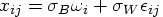, where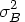is the between-subgroup variance,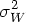is the within-subgroup variance, the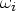are independent with zero mean and unit variance, and theare independent of the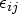. This method can also be used to construct the three-way control chart, which is advocated for this situation by Wheeler (1995). A three-way control chart is useful when sampling, or within-group, variation is not the only source of variation, as discussed in "Multiple Components of Variation" . A three-way control chart comprises a chart of subgroup means, a moving range chart of the subgroup means, and a chart of subgroup ranges. When you specify the SMETHOD=MVGRANGE option, the XRCHART statement produces the appropriate charts of subgroup means and subgroup ranges.
• The TESTLABBOX option produces labels for subgroups with positive tests for special causes that are positioned so they do not overlap, if possible. The labels are enclosed in boxes which are connected to the associated subgroup points with line segments.
• The TESTSYM= option specifies a symbol for plotting subgroups with positive tests for special causes.
• The TESTSYMHT= option specifies the height of the symbol used to plot subgroups with positive tests for special causes.
• The WNEEDLES= option specifies the width, in pixels, of needles that connect plotted points to the central line.
•The ZEROSTD=NOLIMITS option suppresses the degenerate control limits on a control chart produced when the estimated process standard deviation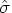is zero.

## References

Wheeler, D. J. (1995), Advanced Topics in Statistical Process Control, Knoxville, TN: SPC Press, Inc.